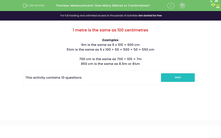# Convert between metres and centimetres

In this worksheet, students convert between centimetres and metres.Key stage:  KS 2

Curriculum topic:   Measurement

Curriculum subtopic:   Measure, Compare, Add/Subtract Lengths, Mass, and Volume/Capacity

Difficulty level:#### Worksheet Overview

In this activity, we will be learning to convert between centimetres and metres.

In maths, we shorten units of measure to make it quicker to record our work. Remember these short versions of the units.

metre = m

centimetre = cm

1 metre is equal to 100 centimetres.

Half a metre is equal to 50 centimetres.

To convert between metres and centimetres there are some simple rules we can use to convert correctly.

This diagram shows how to multiply and divide by 100 to convert cm and m.6m is the same as 6 x 100 = 600cm

5½m is the same as 5 x 100 + 50 = 500 + 50 = 550cm

700cm is the same as 700 ÷ 100 = 7m

850cm is the same as 8.5m or 8½m

Let's try an example question.

Example 1

How many centimetres are equal to two and a half metres?

We know that 2m = 200 cm.

We also know that half a metre is 50cm.

200 + 50 = 250

So, two and a half metres is equal to 250cm.

Example 2

How many metres are equal to six hundred centimetres?